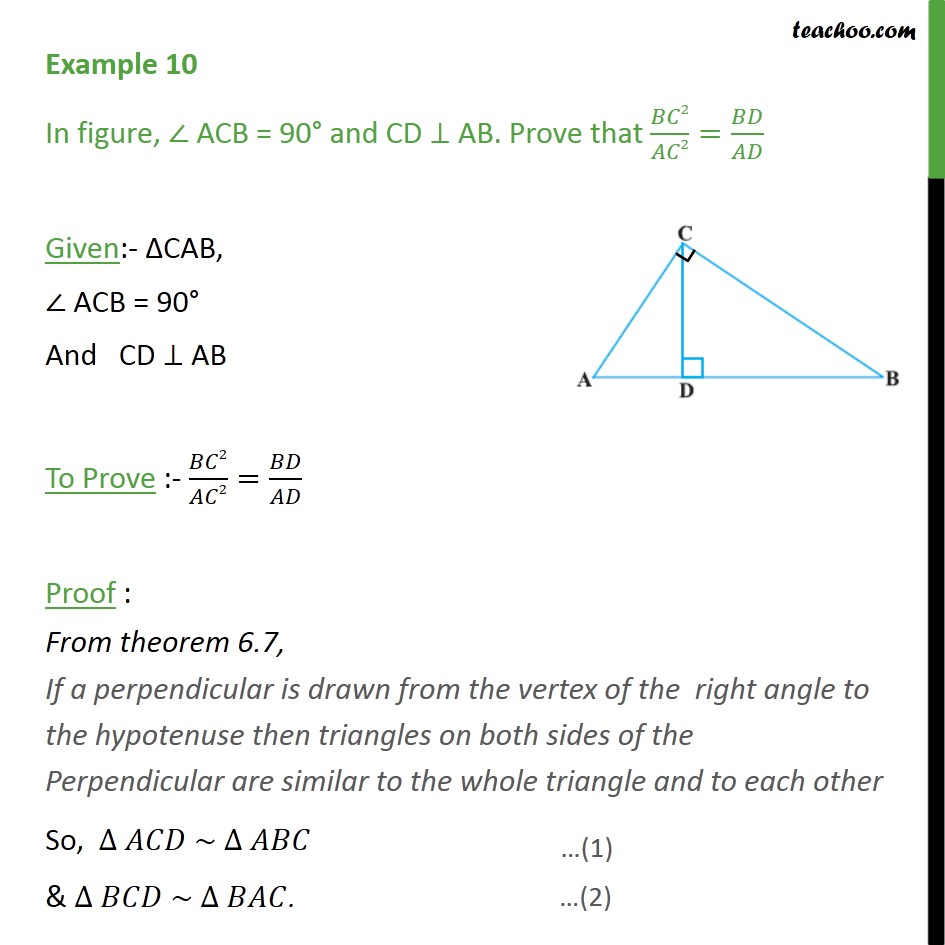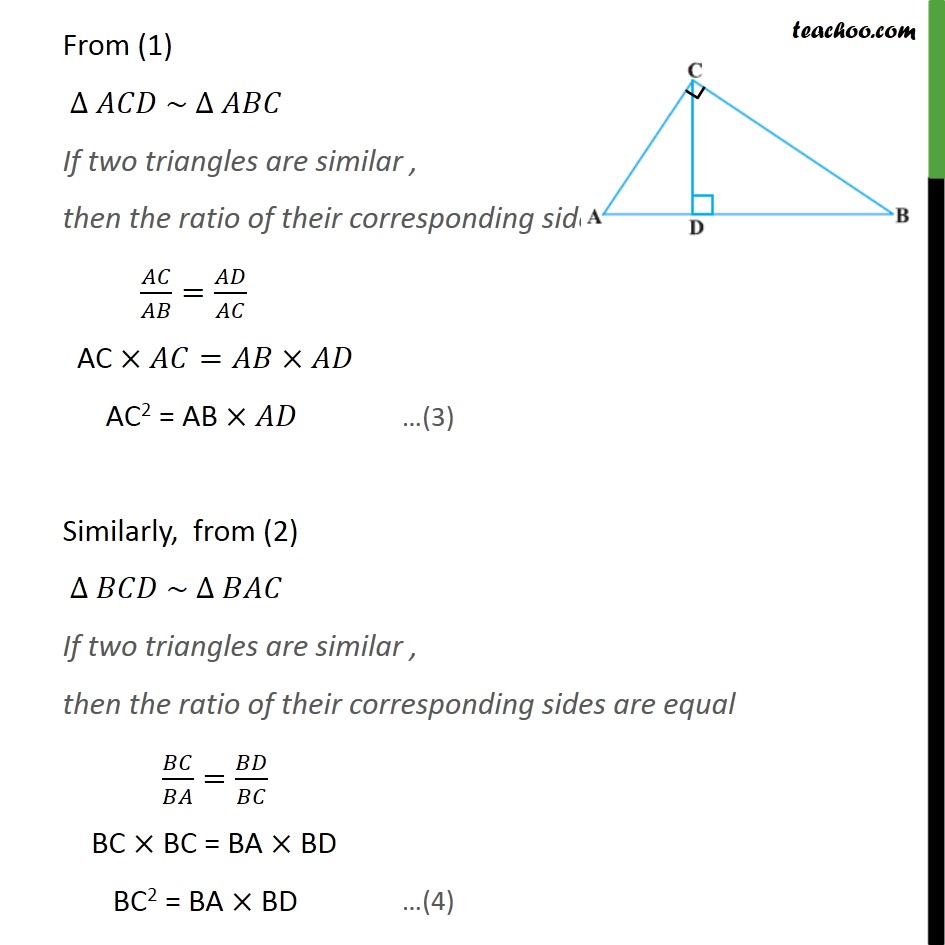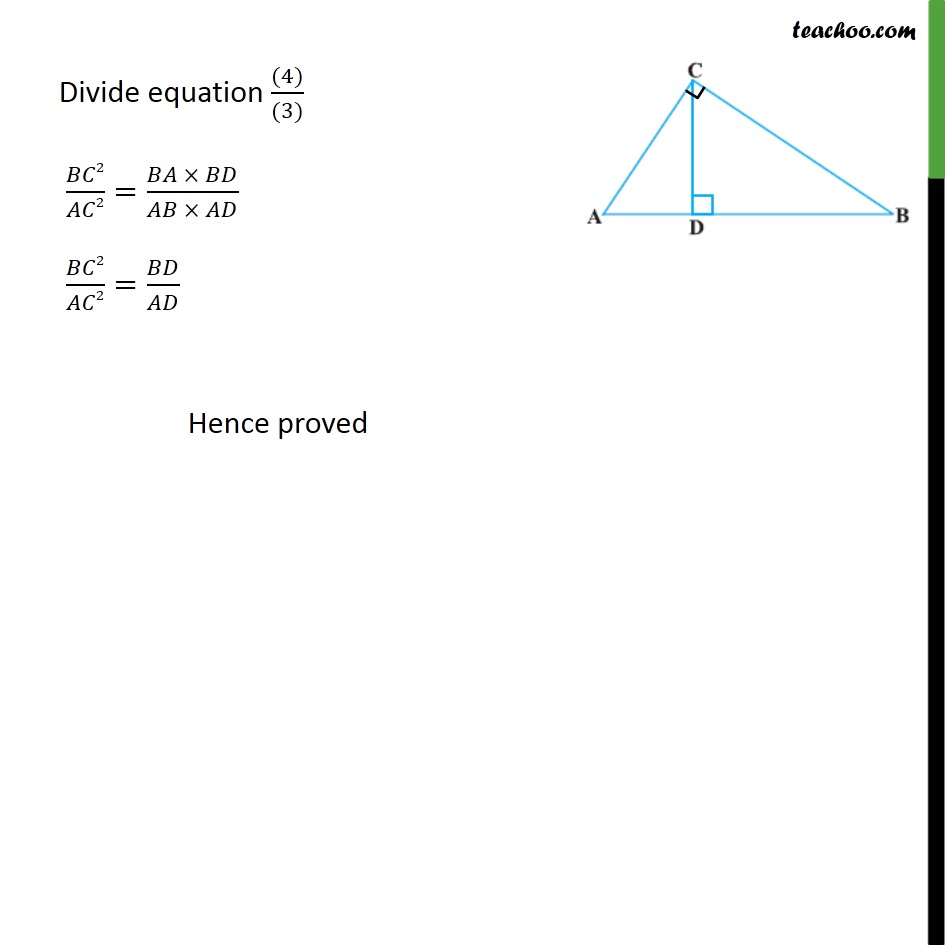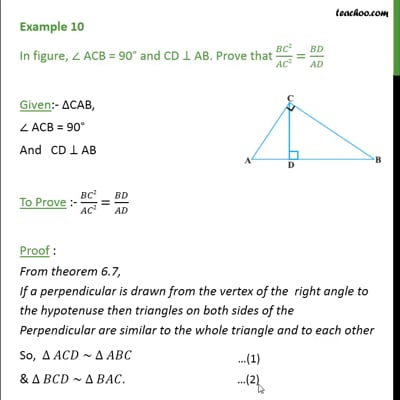Examples

Chapter 6 Class 10 Triangles
Serial order wiseThis video is only available for Teachoo black users

Maths Crash Course - Live lectures + all videos + Real time Doubt solving!

### Transcript

Example 10 In figure, ∠ ACB = 90° and CD ⊥ AB. Prove that BC2/AC2=BD/AD Given:- ΔCAB, ∠ ACB = 90° And CD ⊥ AB To Prove :- BC2/AC2=BD/AD Proof : From theorem 6.7, If a perpendicular is drawn from the vertex of the right angle to the hypotenuse then triangles on both sides of the Perpendicular are similar to the whole triangle and to each other So, ∆ 𝐴𝐶𝐷 ~ ∆ 𝐴𝐵𝐶 & ∆ 𝐵𝐶𝐷 ~ ∆ 𝐵𝐴𝐶. From (1) ∆ 𝐴𝐶𝐷 ~ ∆ 𝐴𝐵𝐶 If two triangles are similar , then the ratio of their corresponding sides are equal 𝐴𝐶/𝐴𝐵=𝐴𝐷/𝐴𝐶 AC ×𝐴𝐶=𝐴𝐵×𝐴𝐷 AC2 = AB ×𝐴𝐷 Similarly, from (2) ∆ 𝐵𝐶𝐷 ~ ∆ 𝐵𝐴𝐶 If two triangles are similar , then the ratio of their corresponding sides are equal 𝐵𝐶/𝐵𝐴=𝐵𝐷/𝐵𝐶 BC × BC = BA × BD BC2 = BA × BD Divide equation ((4))/((3)) 𝐵𝐶2/𝐴𝐶2=(𝐵𝐴 × 𝐵𝐷)/(𝐴𝐵 × 𝐴𝐷) 𝐵𝐶2/𝐴𝐶2=𝐵𝐷/𝐴𝐷 Hence proved